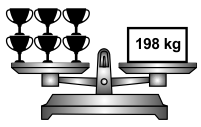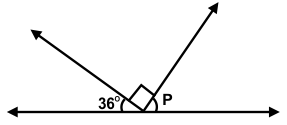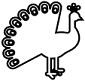# CREST Mathematics Olympiad Class 5 Sample Papers

REGISTER NOW

## Syllabus:

Section 1: Numerals, Number Names and Number Sense (7 and 8 digit numbers), Computation Operations, Fractions and Decimals, Measurement of Length, Weight, Capacity, Volume, Time, Temperature and Money, Conversions, Geometrical Shapes and Solids, Angles, Perimeter of Various Shapes & Area of Rectangle and Square, Symmetry, Data Handling.

Achievers Section: Higher Order Thinking Questions - Syllabus as per Section 1

 Q.1 Choose the correct option: Consider the following statements:A. The sum of two prime numbers is always a prime number.B. The product of two prime is a prime number.Which of these statements is/are correct?
 Q.2 On the basis of the given figure, few statements are made. Choose the correct option with respect to the statements:Statement 1: The weight of 3 trophies is 66 kg more than the weight of 1 trophy.Statement 2: The weight of 4 trophies is more than 150 kg.Q.3 Find the measure of ∠p in the given figure:Q.4 Which of the given options show how the given figure would like if it is quarterly rotated in the clockwise direction?Q.5 If A*B = AB(A - B), then find the value of 509*218:
 Q.6 Which of the following statements is not true?
 Q.7 The simplified value of [18 ÷ 6 + {2 x (8 - 7 of 3) + 17 x 5}] is:
 Q.8 Which of the following figures has maximum lines of symmetry?
 Q.9 The mixed fraction 5 4/7 can be expressed as:
 Q.10 Which of the given shapes can have only 3 lines of symmetry?

Sample PDF of CREST Mathematics Olympiad for Class 5: